American Journal of Theoretical and Applied Statistics
Volume 4, Issue 3, May 2015, Pages: 178-184

Modeling Road Traffic Accident Injuries in Nairobi County: Model Comparison Approach

Julius Nyerere Odhiambo, Anthony Kibira Wanjoya, Anthony Gichuhi Waititu(J. N. Odhiambo)

Julius Nyerere Odhiambo, Anthony Kibira Wanjoya, Anthony Gichuhi Waititu. Modeling Road Traffic Accident Injuries in Nairobi County: Model Comparison Approach. American Journal of Theoretical and Applied Statistics. Vol. 4, No. 3, 2015, pp. 178-184. doi: 10.11648/j.ajtas.20150403.24

Abstract: Road Traffic Accident (RTA) injuries, is a neglected cause of death and disability in Nairobi County. Nairobi County has the highest number of injury rates in Kenya, notably in the active age group of (15-29) years that constitutes approximately 40% of its population. This signifies the importance of properly analyzing traffic accident data and predicting injuries, not only to explore the underlying causes of RTA injuries but also to initiate appropriate safety and policy measures in the County. Thus the study modeled RTA injuries that occurred from 2002 to 2014 in Nairobi County using the Artificial Neural Networks (ANN). ANN is a powerful technique that has demonstrated considerable success in analyzing historical data to predict future trends. However the use of ANN in accidents analysis was found to be relatively new and rare and thus the negative binomial regression approach was utilized as the study’s baseline model. The empirical study results indicated that the ANN model outperformed the negative binomial model in its overall performance.

Keywords: Road Traffic Accidents, Injuries, Artificial Neural Networks, Negative Binomial, Nairobi

1. Introduction

A Road Traffic Accident according to Garber (2010) is a random event involving a road user that results into property damage, death or injury. Road traffic accidents cause an estimated 13 million deaths and 20-50 million disabilities worldwide annually, notably 85% of injury related deaths occur in developing countries. The burden attributed to road safety is comparable with tuberculosis and malaria; approximately it costs 3% of the world GDP. The annual losses in developing countries occasioned by RTAs exceed the annual development aid loans received by these countries (World Bank, 2010). According to WHO (2007), RTA injuries accounted for 23% of all injury deaths worldwide. Nantulya and Muli (2009) argue that road traffic injuries will become the fifth leading cause of death by 2030 if no action is taken.

In Kenya, the road transport sector accounts for over 93% of the total domestic freight and passenger traffic. The road transport infrastructure represents a significant portion of the government’s total investments in fixed assets (KRB, 2012). At independence (1963) the number of deaths from RTA in Kenya was 548. 45 years later the number rose to approximately 3158, a 476% increase of the total number of accidents (Ogendi, 2013). The estimated annual economic cost of road traffic injuries in 1984, applying the human capital approach method, was approximated to be U.S. \$ 14 million, an equivalent of 1.6% of Kenya’s gross national product (GNP). The cost was approximately U.S. \$ 35 million in 1996. This translated into a loss of 26–52% of the total earnings from road transport (Odero, 2003).

Nairobi County is the most populous in East Africa, with an estimated current population of about 3.5 million (KNBS, 2014). Its roads are reported to be the world’s fourth most congested (IBM, Commuter Pain Survey, 2011). According to the Nairobi Traffic Police (2014), it has the number of RTA incidences in the Kenya and of the 3000 people are killed and 12500 seriously injured Nairobi County accounts for over 50% (WHO, 2012).

These alarming statistics underpins the importance of updating and improving accident data records and subsequently the methods of analyzing traffic data as this will help policy makers to formulate evidenced based regulations and road safety measures. Thus this study seeks to develop an artificial neural network model and comparatively measure its performance against the negative binomial model.

2. Review of Previous Research

Researchers have modeled traffic accidents from a highway safety point of view, neglecting the key accident injury contributory factors. Abdelwahab et al (1997) studied accident data from Central Florida focusing on two-vehicle accidents that occurred at signalized intersections. The severity of injury was divided into three classes: no recorded injury, disabling injury and possible injury. The performance of an Artificial Neural Network trained by Levenberg-Marquardt algorithm and fuzzy ARTMAP were compared. Results suggested that Artificial Neural Network (ANN) model performed better than the Fuzzy ARTMAP.

Bedard (2002) used the multivariate logistic regression model to determine the independent contribution of crash, driver and vehicular characteristics that lead to increased driver’s fatality risk. Reducing speed, increasing the use of seatbelts and reducing severity incidences attributed to driver-side impacts was found to be preventing fatalities.

Using a multivariate population-based statistical analysis, Evanco (1999) determined the relationship between fatalities and accident notification times. Evanco’s analysis indicated that accident notification time was a significant determinant of the number of accident fatalities occurring on the roadways.

Kim et al (1995) developed a log-linear model to clarify the role of driver characteristics and behaviors in the causal sequence leading to more severe injuries. It was found that driver behaviors of alcohol use and lack of seat belt use greatly increase the odds of more severe crashes and injuries.

Akomolafe (2007) employed Artificial Neural Network using Multilayer perceptron to predict likelihood of accident happening at a particular location between the first 40 kilometers along Lagos-Ibadan Express road.

3. Artificial Neural Network

According to Gichuhi (2008), a neural network is a parallel connection of a set of nodes referred to as neurons. It represents a function of explanatory variables which is composed of simple building blocks and which may be utilized to provide an approximation of the conditional expectations or, in particular, probabilities in regression. ANN are capable of approximating any finite non-linear models so as to determine the relation between dependent and independent variables. Notably in ANN no assumptions are required concerning the functional form of the relationship existing between predictor and response variables as is the case with other statistical models.

3.1. Multilayer Perceptron

A neural network system is based on a unit called a perceptron. A multi-layer perceptron is a feed forward ANN that maps sets of input data onto a set of outputs.

The design and the training of a multilayer perceptron network involves challenges, which include determining the number of hidden layers to be used in the network, determining the number of neurons to be used in each hidden layer, establishing a general acceptable solution that avoids local minima, converging to an optimal solution in good time, and validating the neural network to test for over fitting.

3.2. Developing the Artificial Neural Network Model

The study considered a feed- forward network with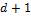input nodes, one layer of H hidden nodes, one output node and an activation function 𝝍(x).

According to Nelson (1991), the success in designing a neural network depends on a clear understanding of the problem. In an attempt to produce an ANN with the highest predictive power, the following networks inputs were as follows;

Ÿ Dependent variable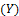representing the number of Injuries

Ÿ Independent variables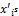which included drivers, pedestrians, pedal cyclists, passengers, animals, obstruction, vehicle defect, road defects and weather.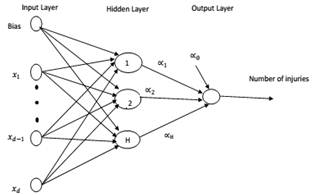Figure 1. Architecture of the ANN model.

We have d-input nodes, one layer of H-hidden nodes and an activation function 𝝍(x).

Input at hidden layer nodes are connected by weights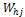forand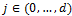.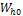is the bias for the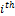hidden node.

The hidden and output layers are connected by weights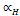forConsidering an input vector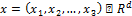.

The input vector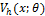to the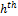hidden node is: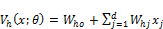(1)

The output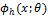of thenode is: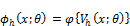(2)

The net-input to the output node is: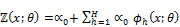(3)

The output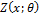of our neural network is: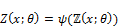(4)

𝜃 stands for all the parameters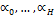and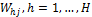and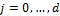of our neural network. We can also write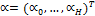and3.3. Artificial Neural Network Training

The connecting weights in an artificial neural network are adjusted through training. Training can either be supervised or non-supervised. Supervised training of a neural network as was employed in this study demanded the following specifications.

Ÿ A sample of n input vectors,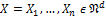of size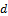each and an associated output vector,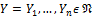Ÿ Selection of an initial weight set.

Ÿ A repetitive method to update the current weight of the network so as to optimize the networks input-output map

Ÿ A stopping rule.

There are two methods used to train a neural network, namely the maximum likelihood estimator (MLE) method and the sum of squared errors (SSE) method. The error function chosen depends on the conditional distribution of the training data. Mitchell (1997) argues that the sum of squares error method is efficient in training multi-layered perceptron neural network as was used in the study.

The sum of squares error (SSE) is defined as: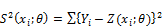(5)

Where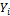is the target output of the neuron, and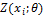is the actual output of the neuron

An important step in training the neural network shall involve updating the neuron weights until the error function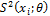is minimized.

There are various method of minimizing the error function namely back propagation, quasi newton method and simulated annealing method. The back propagation approach will be used in this study.

3.4. Back Propagation (BP)

The back-propagation in the study uses the gradient descent training algorithm. This algorithm adjusts the weights as it moves down the steepest slope of the error surface i.e. it is considered to have converged when the Euclidean norm of the gradient vector reaches a sufficiently small gradient threshold.

By taking a unipolar activation function, the weights are adjusted as: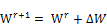(6)(7)

By taking individual weights, we have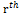iteration weights as: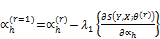(8)

for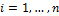and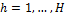Similarly;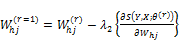(9)

forandand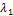andrepresents the step gain.

The weights are to be adjusted until the stopping criterion is met. Each weight is adjusted n times at each iteration. This means that foriterations, each weight is adjustedn times.

Notably the weights of the ANN are to be adjusted to enable the ANN approximate the target function with sufficient precision. The simplest way to stop the training is to limit the number of iterations to a predetermined value. This stopping criterion is frequently used mainly when a new problem is solved and nothing is known about the shape and properties of the error surface (White, 1989).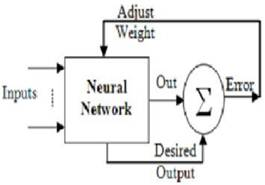Figure 2. Artificial neural network learning model.

4. Negative Binomial Regression

The Poisson regression model is often referred to as the benchmark model for modeling count data. It dominates the count data modelling activities as it suits the statistical properties of count data and is flexible for it can re-parameterized into other form of distribution functions (Cameron and Trivedi, 2013). The negative binomial is a distribution that is concentrated on the non-negative integers, unlike the poisson distribution; it has an additional parameter that provides for the variance to exceed the mean.

The Poisson regression model assumes a log-linear relationship between the poisson parameter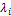and explanatory variables.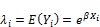(10)

Where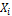is a vector of explanatory variables and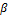is a vector of unknown regression coefficients.

The negative binomial regression relaxes the assumption of equality of the mean and variance. By adding a gamma -distributed error term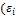) is rewritten as,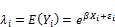(11)

The error term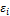is gamma-distributed with mean 1 and variance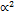.The addition ofmakes the variance to be different from the mean as follows: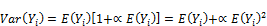(12)

Where,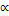is the dispersion parameter.

When the dispersion parameterapproaches zero, the variation is almost equal to mean, and the distribution can thus be modeled using the poisson regression technique.

The primary equation of the negative binomial model is: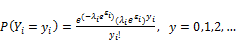(13)

5. Methodology

5.1. Selection of Input Variables

Spearman's rank correlation coefficient was used as the non-parametric measure of correlation, this was due to its robustness when extreme values are presents. According to Zhang (1998), correlated input variables may worsen the prediction performance by interacting with each other and generating a biased effect.

5.2. Data Preprocessing

Data preprocessing assists the neural network in learning the relevant patterns, which subsequently improves the data fitting and prediction accuracy. The sigmoid activation function was used in the study's neural network. The sigmoid function had an upper bound of one and a lower bound of zero. Thus the ANN input variables had to be transformed into the range of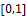.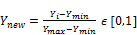(14)

Where,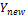denotes the transformed variable,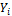denotes the observed value of variable,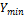denotes the minimum value of the input variable and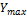denotes the maximum value of the input variable.

5.3. Number of Hidden Layers

The number of hidden layers in a neural network provides a network with an ability to generalize. Increasing the number of neural networks increases the computational time and increases the chance of over-fitting, as this may force the network to memorize as opposed to generalize. This study adopted a neural network with one hidden layer, as they are widely used and have performed well (Baum and Haussler, 1989).

5.4. Determining the Number of Hidden Nodes

Deciding on the number of nodes in the hidden layer is important as it helps determine the neural network architecture. The study compared different number of nodes with their corresponding goodness of fit value.

The following equation was utilized in determining the number of hidden nodes (Yuen and Lam, 2006)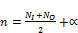(15)

Where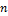the number of hidden nodes is,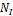is the number of input neurons,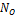is the number of output neurons andwas arbitrarily taken to be 2

Using the dataset, the value of the coefficient of determination was used to determine the optimal number of hidden nodes in our neural network. This study thus settled on seven hidden nodes as shown in figure 3.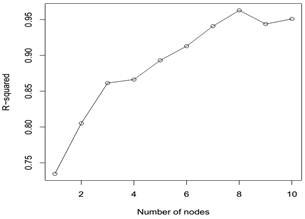Figure 3. Determining the number of hidden nodes.

5.5. Training and Testing Data

The training set ranged from January 2002 to December 2013. The testing set ranged from January 2014 to December 2014. The training set was used to optimize the weights and the bias of the network, while testing was used to indicate the generalization ability of the network.

5.6. Performance Measures

The objective of each of the methods used was to fit an accurate model that was to be used in predicting future injuries. According to Ghaffari (2006) the adequacy of the negative binomial model and artificial neural network is assessed on the basis of mean squared error (MSE), coefficient of determination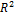and the root mean squared error (RMSE).

An MSE value closer to 0, indicates a fit that is more useful for prediction. The mean squared error was calculated as follows.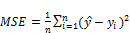(16)

Where,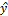denotes the predicted value,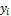denotes the actual value andis the size of the predicting sample.

The non-parametricwas formulated as the within sample, measure of goodness of fit for the artificial neural network.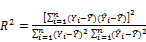(17)

Where,denotes the outcome,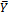denotes the sample mean and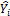denotes the fitted value of observation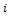.

6. Results and Discussion

6.1. Multicolinearity in Explanatory Variables

Table 1. Correlation matrix result.

 Variable Drivers Pedal- Cyclist Pedestrians Passengers Animals Obstruction Vehicle- Defects Road- Defects Weather Injury Drivers 1.0000 0.2384 0.3149 0.1517 0.0556 0.2097 0.1889 0.0663 0.2352 0.6616 Pedal-Cyclist 1.0000 0.1930 0.2686 -0.0167 0.1642 0.2255 0.4226 0.2178 0.3789 Pedestrians 1.0000 0.3584 0.0265 0.2089 0.1973 -0.0488 0.1602 0.6145 Passengers 1.0000 0.0687 0.2485 0.1185 0.0626 0.0416 0.478 Animals 1.0000 -0.0144 0.0946 0.081 0.0285 0.0479 Obstruction 1.0000 0.2693 0.3616 0.169 0.2769 Vehicle-Defects 1.0000 0.1741 0.146 0.1751 Road-Defects 1.0000 0.2878 0.0883 Weather 1.0000 0.1444 Injury 1.0000

On the strength of the correlation coefficients between variables the result indicated that drivers (0.6616), pedestrians (0.6145) and passengers (0.4780) had the highest correlation to the number of injuries in Nairobi County. This was followed by pedal-cyclists (0.3789), obstruction (0.2769), vehicle-defects (0.1751), and weather (0.1444) respectively. Road-defects (0.0883) and animals (0.0479) were not correlated to the number of injuries. Importantly the study's explanatory variables were not correlated, as their correlation coefficients were less than 0.500.

6.2. Negative Binomial Regression

Table 2. Negative-binomial regression results.

 Estimate Standard Error Z-value Pr (>|z|) Intercept 5.0227 0.06320 79.4810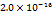Drivers 0.0047 0.0005 9.1470Pedal-Cyclists 0.0048 0.0019 2.5690 0.0102 Pedestrians 0.0026 0.0005 5.3960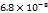Passengers 0.0042 0.0021 2.0060 0.0449 Animal 0.0028 0.0198 0.1410 0.8876 Obstruction 0.0094 0.0088 1.0710 0.2841 Vehicle-Defects -0.0062 0.0059 -1.0540 0.2918 Road Defects 0.0035 0.0173 0.200 0.8418 Weather -0.0152 0.0096 -1.5880 0.1123

The study noted as indicated in table 2, that drivers, pedal cyclists, pedestrians and passengers significantly determined the total number of monthly injury occurrence in Nairobi county.

6.3. Artificial Neural Networks

Table 3. Artificial neural network results.

 Data-set Number of Samples Mean Squared Error Non-parametric R2 value Root mean-squared error Testing 144 0.0040 0.8946 0.0632 Training 12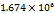0.9998 0.0013

From the results in table 3; for the training data set, 89.46% of the monthly number of RTAs injuries was explained by the network input variables. For the testing data set, 99.97% of RTAs injuries for the year 2014 was explained by the network input variables. The root mean squared error of the testing data set was smaller as compared to the training data set. This observation implies that the testing data set, could be used to generalize the network performance.

6.4. Predictive Comparison of the ANN and Negative Binomial Model

The number of injuries predicted by the negative binomial model and the ANN model were compared with the actual observations and the results are indicated in table 4.

Table 4. Model comparison results.

 Year (2014) Actual Observed ANN Prediction Negative-Binomial Prediction Jan 243 246 262 Feb 227 265 269 March 302 323 299 April 258 262 267 May 336 326 287 June 242 259 273 July 279 280 297 Aug 359 344 338 Sept 259 274 297 Oct 255 297 292 Nov 305 273 284 Dec 255 260 279

The monthly number of road traffic injuries (RTIs) predicted by the ANN model were compared with the actual observed values for the year 2014. The April, July and December ANN predictions differs from the actual prediction by less than 1%. The ANN prediction yielded optimal values when compared to the negative-binomial prediction.

Figure 4, is a line-graph showing the actual number of injuries against the model values across different months in the year 2014. For the negative binomial model, the graph shows marked deviations from the actual observed values. The ANN estimates, on the other hand, are much closer to the actual values.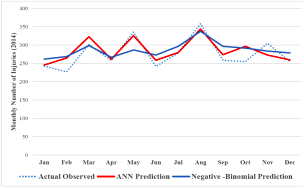Figure 4. Model comparison line-graph.

6.5. Performance Measures

The objective of each of the methods used was to fit an accurate model of the accidents data for use to predict future injuries. The adequacy of the negative binomial model and the artificial neural network is assessed on the basis of MSE, the coefficient of determination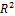and the root mean squared (RMSE).

Table 5. Performance measures results.

 Model Mean-Squared Error (MSE) Root Mean-Squared Error Negative Binomial 0.6691 148.3875 12.1814 Artificial Neural Network 0.8946 0.0040 0.0632

From the results in table 5, artificial neural network technique outperforms the negative binomial regression technique, since it had the minimal values of the mean-squared error and the root mean squared. Its coefficient of determination value was 0.8946 which was greater than 0.6691. This implied that for the ANN model 89.46% of RTA injuries could be explained by our independent variables, whereas for the negative binomial model 66.91% of RTA injuries could be explained by our independent variables.

7. Conclusion

Artificial neural network was used to model the monthly number of road traffic injuries and the negative binomial regression model as our baseline model. The study noted that accident data are non-negative integers, and thus the application of standard ordinary least-squares regression (which assumes a continuous dependent variable) was not appropriate.

The artificial neural network generalization ability, outperforms the negative binomial regression in its overall performance and thus should be adopted as the technique for predicting monthly road traffic injuries.

Future research should concentrate on the spatial modeling of RTAs injuries in Nairobi County and then scale up to the whole of Kenya. Accident prediction models should extend and take into account the combined effect of different explanatory variables.

References

1. Abdel-Aty, M. A. and H. T. Abdelwahab (2004). Predicting injury severity levels in traffic crashes: a modeling comparison. Journal of transportation engineering 130 (2), 204–210.
2. Abdelwahab, H. T. and M. A. Abdel-Aty (2001). Development of artificial neural network models to predict driver injury severity in traffic accidents at signalized intersections. Transportation Research Record: Journal of the Transportation Research Board 1746 (1), 6–13.
3. Akomolafe, D. T., F. Adekayode, J. Gbadeyan, and T. Ibiyemi (2009). Enhancing road monitoring and safetythrough the use of geospatial technology. International Journal of Physical Sciences 4 (5), 343–348.
4. Bayata, H. F., F. Hattatoglu, and N. Karsli (2011). Modeling of monthly traffic accidents with the artificial neural network method. Int J Phys Sci 6, 244–54.
5. Bedard, M., G. H. Guyatt, M. J. Stones, and J. P. Hirdes (2002). The independent contribution of driver, crash, and vehicle characteristics to driver fatalities. Accident Analysis & Prevention 34 (6), 717–727.
6. Cameron, A. C. and P. K. Trivedi (2013). Regression analysis of count data, Volume 53. Cambridge university press.
7. Evanco, W. M. (1999). The potential impact of rural mayday systems on vehicular crash fatalities. Accident Analysis & Prevention 31 (5), 455–462.
8. Gaber, S., F. (2010).Analysis and Assessment of Accident Characteristics: Case Study of Dhofar Governorate, Sultanate of Oman, International Journal of Traffic and Transportation Engineering, 3(4), 189-198.
9. IBM, Commuter Survey (2014).
10. Kenya Bureau of Statistics (2014). Statistical Abstract
11. Kenya Roads Board (2012). Annual Report
12. Kim, K., L. Nitz, J. Richardson, and L. Li (1995). Personal and behavioral predictors of automobile crash and injury severity. Accident Analysis & Prevention 27 (4), 469–481.
13. Manyara, C. G. (2013). Combating road traffic accidents in Kenya: A challenge for an emerging economy. In Proceedings from the KESSA 2013 Conference, pp. 6–7.
14. Mitchell, T. M. (1997). Machine learning. 1997. Burr Ridge, IL: McGraw Hill 45.
15. Nairobi Traffic Police Department (2014).
16. Nantulya, V. M. and M. R. Reich (2002). The neglected epidemic: road traffic injuries in developing countries.BMJ: British Medical Journal 324 (7346), 1139.
17. Nelson, M. M. and W. T. Illingworth (1991). A practical guide to neural nets, Volume 1. Addison-Wesley Reading, MA.
18. Odero, W. (1995). Road traffic accidents in kenya: an epidemiological appraisal. East African medical journal 72 (5),299–305.
19. Odero, W. (1997). Kenya: road-traffic accidents. The Lancet 349, S13.
20. Odero, W., P. Garner, and A. Zwi (1997). Road traffic injuries in developing countries: a comprehensive review of epidemiological studies. Tropical Medicine & International Health 2 (5), 445–460.
21. Odero, W., M. Khayesi, and P. Heda (2003). Road traffic injuries in kenya: magnitude, causes and status of intervention. Injury control and safety promotion 10 (1-2), 53–61.
22. World Health Organization. (2014). World health statistics.Retrieved fromA989?lang=en
23. World Health Organization (2013). Global status report on road safety 2013: Supporting a decade of action.
24. WHO (World Report on Road Traffic Injury Prevention) (2012).
25. Yuen, K.-V. and H.-F. Lam (2006). On the complexity of artificial neural networks for smart structures monitoring.Engineering Structures 28(7), 977–984.
26. Zhang, G., B. E. Patuwo, and M. Y. Hu (1998). Forecasting with artificial neural networks:The state of the art. International journal of forecasting 14 (1), 35–62.

 Contents 1. 2. 3. 3.1. 3.2. 3.3. 3.4. 4. 5. 5.1. 5.2. 5.3. 5.4. 5.5. 5.6. 6. 6.1. 6.2. 6.3. 6.4. 6.5. 7.
Article ToolsAbstractPDF(333K)